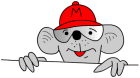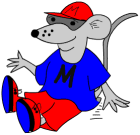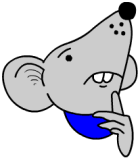## Booster maths worksheet 1

They are all pages of questions of the kind found on the test papers, starting with some basic four rules questions.This is the first in my series of ‘Booster’ worksheets for year 6 children who are about to take the SAT tests. They aim to boost a child’s score in the test papers by making them familiar with the types of questions that they will come across.

It is rare for a straightforward sum of the type 34 + 67 = to appear on the paper. Usually sums are in the form of number sentences with numbers missing. Often there can be more than one correct answer.

For example, if you look at the first question, there are many possible answers. A tip here is to keep it simple. Why not write 46 + 10? Perfectly correct!

Booster maths worksheet 1

## Reception vocabulary: Read and write numbers to 20

The free maths worksheets below goes to the SECOND in a list of the words and phrases to do with counting that children will be expected to understand and use by the end of their Reception Year, according to the Primary Framework for Mathematics.

The free maths worksheet below goes to the SECOND in a list of the words and phrases to do with counting that children will be expected to understand and use by the end of their Reception Year, according to the Primary Framework for Mathematics.
COUNTING FROM 10 TO 20 Continue reading “Reception vocabulary: Read and write numbers to 20”

## Maths Puzzle: Well it is Christmas!Looking at my stats I can see that everyone is very busy with shopping, eating, drinking etc so will join you all and have a week off. I will leave you with a little puzzle in case you have nothing better to do!

Put the numbers 1, 2, 3, 4, 5 in the bottom layer of baubles on the Christmas tree. The next layer is made by adding the pairs of numbers below and so on up to the top. Continue reading “Maths Puzzle: Well it is Christmas!”

## Maths game: Count back in ones (Teddies)

A little maths game for helping with counting back from single digits.

A little maths game for helping with counting back from single digits.

The sun’s shining and it’s hot. Teddy’s gasping for a drink. Can you help him finish his counting back?

## Free Y3 maths worksheet: Make number stories: division

By Year 3 children should also be recognising sharing or division is the inverse of multiplication.One of the key elements in division is to recognise that a number of objects needs to be shared equally. To begin with this can be done on a ‘one for you, one for me’ basis. Some children become fixed on this and always think of division as sharing into 2. Therefore, it can still be useful to use practical objects to share into a number of groups, making sure that each group has an equal number in it. Continue reading “Free Y3 maths worksheet: Make number stories: division”

## Free Y2 maths worksheet: Making number stories: division

This maths worksheet helps to show whether a child has understood divisionAnother worksheet for our ‘Using and Applying Maths’ strand. Most children have more difficulty with division than any of the other operations. At this early stage it is important to try and get across the idea of division as sharing. This can be done in a practical way with sweets, coins etc. This maths worksheet helps to show whether a child has understood division by asking them to make up their own sentences having been given the numbers. Continue reading “Free Y2 maths worksheet: Making number stories: division”

## Reception vocabulary: Read and write numbers to 10

zero, one, two, three, four, five, six, seven, eight, nine, ten

The following pages give some practice at reading and writing these words. Use the light grey letters to trace over and the lines to control the size of the letters.

This is the first of a series looking at the words and phrases that children will be expected to understand and use by the end of their Reception Year. (According to the Primary Framework for Mathematics.)

We start by looking at numbers to ten.

zero, one, two, three, four, five, six, seven, eight, nine, ten

The following pages give some practice at reading and writing these words. Use the light grey letters to trace over and the lines to control the size of the letters. Continue reading “Reception vocabulary: Read and write numbers to 10”

## Free Y6 maths worksheet: Revise understanding subtraction

High-quality direct teaching is oral, interactive and lively. It is not achieved by adopting a simplistic formula of ‘drill and practice’, or by expecting pupils to teach themselves from books.“High-quality direct teaching is oral, interactive and lively. It is not achieved by adopting a simplistic formula of ‘drill and practice’, or by expecting pupils to teach themselves from books. It is a two-way process in which pupils are expected to play an active part by answering questions, contributing points to discussions, and explaining and demonstrating their methods to the class.”

(From the Primary Framework for Mathematics.)

The importance of this kind of work is clearly stated above. Don’t just give this maths worksheet to your child, go through it with them, help them use the language of maths to secure their recall, thinking and reasoning skills.

Free Y6 maths worksheet: Revise understanding subtraction (pg 3)

## Free Y5 maths worksheet: Revise understanding subtractionVery similar to the Year 4 worksheet sent up yesterday. By now children should be checking answers by using the reverse operation as a matter of course. Unfortunately, like most of us they probably don’t!

Encourage them to do this as it is far less likely to lead to errors if there is not a calculator handy to check the answer.

Free Y5 maths worksheet: Understand subtraction and its relationship to addition (pg 3)

## Free Y4 maths worksheet: Understand subtraction and its relationship to additionThis free maths worksheet looks at the relationship between addition and subtraction, in particular, that subtraction is the inverse or opposite of addition. This gives us an excellent way to check answers.

Look at 450 – 51.

We can do the subtraction sum and then check by doing the same subtraction sum again. But there is a danger with this that we might make the same mistake twice.

A better way is to do the subtraction and get the answer (399) and then check by adding the answer 399 to 51 which gives 450. Yes that is the same as the question.

Free Y 4 maths worksheet: Understand subtraction and its relationship to addition (pg 3)